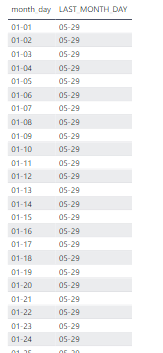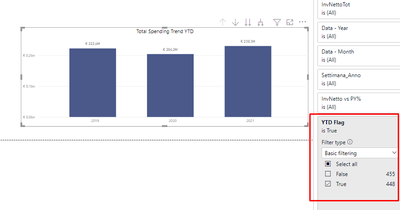cancel
Showing results for
Did you mean:## SAMEPERIODLASTYEAR with YearToDdate filter based on This Year

Since I've had some trouble finding a solution to my problem in the community, I write this solution that might help somebody.

In this thread, I show my solution on how to obtain a Comparable period based on DATES of this year. Usually, in the real life, you don't have a full period this year to make a trend/comparison in a chart with previous year periods:in the chart above, 2021 sales refer only to  01.Jan - 31.May period while the 2020 column refers to whole 2020.

What I wanted to show is the only 01.Jan - 31.May of 2020 period in order to have the "sameperiodlastyear" calculation for each year in the chart.

The problem of using "SAMEPERIODLASTYEAR" function is that it automatically consider the actual context and switch to the previous similar period of the specific context. this means that in the chart, under 2020 column you would see the whole previous 2019 period instead of Jan-May.

Here is my solution:

basically we will set "FlagYTD" column in our datetable that we will use in our measuers or as a chart filter. This will be based on "month-day" so that for each year we will consider only days before the last day of this year.

1) first of all you need to create the "month-day" column in your DATETABLE (you can use also your fact-table):

``````month_day =
var monthN=DATETABLE[Data].[MonthNo]
var dayN=DATETABLE[Data].[Day]
return
if(len( monthN)=1, "0","")&monthN
&"-"&
IF(len(dayN)=1, "0","")&dayN``````

this is what you should get- adding leading zeros at the beginning allows the correct alphabetical order of month-days columns that can be used in a string comparison (<, >,)

2) at this point we will identify the last "month-day" of this year in the SALES / FACT table:

``````LAST_MONTH_DAY=
var lastday = calculate(max(SALESTABLE[Date]), all(SALESTABLE))
var monthN=month(lastday)
var dayN= day(lastday)
return
if(len( monthN)=1, "0","")&monthN
&"-"&
IF(len(dayN)=1, "0","")&dayN``````

and this is the two created columns together (in my case the last date in my dataset for this year is 29 may 2021):3) we can now create the "FLAG_YTD" column:

``YTD Flag = DATETABLE[month_day]<=[LAST_MONTH_DAY]``

Now you can either use the FLAG for calculated columns such as:

``SALES_YTD= CALCULATE([InvNettoTot],CalDay_INV[YTD Flag])``

and use it as a double value column_chart or for any Comparison formulas (this can be used to calculate %detlas between periods )Or maybe you can just filter out your chart (using the sum(SALES) measure) and obtain:For me this was a very helpful trick that helped me out with lots of very useful charts and comparison.

i hope this will help someone of you too.

-francesco

2 REPLIES 2Community Support

Hi, @fsolarino

So that other community members will easily find the solution when they get the same issue.

Best Regards,
Community Support Team _ EasonSuper User

@fsolarino , Based on what I got try a measure like this example

LYTD Corrected =
var _max = format(today(),"MMDD")
return

You can use maxx selected date inplace of today